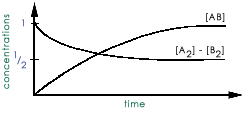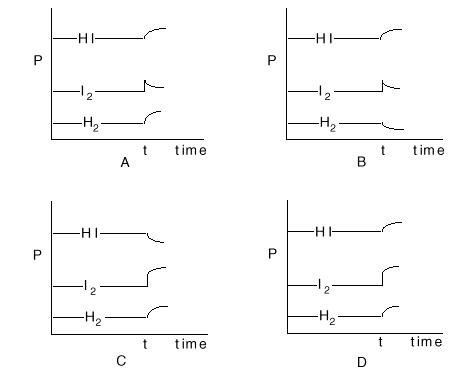# Chemical Equilibria Concept Test

## Problem 1

Consider the reaction: A2 + B2 <---> 2AB The reactants are mixed together and the following is a plot of the reactant and the product concentrations with time:what is the value of the equilibrium constant?

1. 1/2
2. 1
3. 2
4. 4

## Problem 2

From a chemical handbook you find the equilibrium constants that give the concentrations of Iodine vapor above water and oil: I2(water) <---> I2(g)    Kwater I2(oil) <---> I2(g)         Koil But you want the equilibrium constant for the partitioning of I2 between water and oil: I2(water) <---> I2(oil)  K You can obtain it as:

1. K = Kwater + Koil
2. K = KwaterKoil
3. K = Kwater/Koil
4. K = Koil/Kwater

## Problem 3

Consider the reaction: N2 + 3H2 <---> 2NH3 The equilibrium constant at 400C is K=0.5. Suppose we make a mixture with the following concentrations: [ NH3] = 1.0M [ N2] = 1.0M [ H2] = 1.0M In which direction will the reaction go?

1. N2 + 3H2 <---> 2NH3
2. 2NH3 --> N2 + 3H2

## Problem 4

For the reaction: N2 + 3H2 <---> 2NH3 The relationship between Kc and Kp is:

1. Kp = Kc(RT)-2
2. Kp = Kc(RT)-1
3. Kp = Kc(RT)1
4. Kp = Kc(RT)2

## Problem 5

Consider the equilibrium expression for the following reaction: CaCO3(s)<---> CaO(s) + CO2(g) Which of the following is true?

1. The ratio of CaO(s) to CaCO3(s) will be the same in all samples of this solid.
2. The vapor pressure of CO2(g) above a mixture of CaCO3(s)/CaO(s) is independent of the relative amount of these two solids.

## Problem 6

The cobalt complexes participating in the equilibrium below comprise a humidity sensor.  From Le Chatelier's principle, when the air is moist, what color is the cobalt complex? Co(H2O)6+2 + 4Cl- <---> CoCl4-2 + 6H2O pink                              blue

1. Pink
2. Blue
3. White
4. Black

## Problem 7

If the density of graphite is about 2 g/ml and that of buckyball is about 1.5 g/ml, in which direction would the application of high pressure drive the equilibrium?

1. toward formation of graphite
2. toward formation of buckyball
3. neither would be formed preferentially

## Problem 8

Consider the gaseous equilibrium: H2(g) + I2(g)<---> 2HI(g) The sketches below show the pressures, P, of these three gases as a function of time during an experiment. The system is initially at equilibrium. At some time, t, extra I2 is added. Which of the sets of curves shows how the system will respond to this situation?